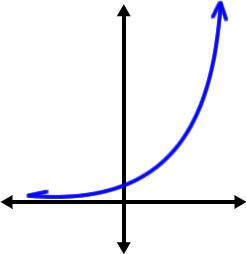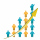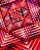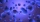# Geometric progression

In geometric progression, a1 = 7, q = 5. Find the condition for n to sum first n members is sn≤217.

n =  3

### Step-by-step explanation:Did you find an error or inaccuracy? Feel free to write us. Thank you!## Related math problems and questions:

• Two geometric progressionsInsert several numbers between numbers 6 and 384 so that they form with the given GP numbers and that the following applies: a) the sum of all numbers is 510 And for another GP to apply: b) the sum of entered numbers is -132 (These are two different geome
• Exponential equationFind x, if 625 ^ x = 5 The equation is exponential because the unknown is in the exponential power of 625
• Geometric sequence 3In geometric sequence is a8 = 312500; a11= 39062500; sn=1953124. Calculate the first item a1, quotient q, and n - number of members by their sum s_n.
• Sum of the seventeen numbersThe sum of the 17 different natural numbers is 154. Determine the sum of the two largest ones.
• Three members GPThe sum of three numbers in GP (geometric progression) is 21 and the sum of their squares is 189. Find the numbers.
• Five elementThe geometric sequence is given by quotient q = 1/2 and the sum of the first six members S6 = 63. Find the fifth element a5.
• MicroorganismsThe first generation of micro-organisms has a population of 13500 members. Each next generation is 11/10 times the previous one. Find out how many generations will reach at least three times members of the first generation.
• Geometric sequenceIn the geometric sequence is a4 = 20 a9 = -160. Calculate the first member a1 and quotient q.
• CrystalThe crystal grows every month 1.2 permille of its mass. For how many months to grow a crystal from weight 177 g to 384 g?
• DemographicsThe population grew in the city in 10 years from 30000 to 34000. What is the average annual percentage increase of population?
• GP - three membersThe second and third of a geometric progression are 24 and 12(c+1), respectively, given that the sum of the first three terms of progression is 76. determine the value of c.
• To the cinemaJane at 8 am got message that all 1093 school pupils will go to the cinema. Within 20 min she said it to the three friends. Each of them again for 20 minutes said to the other three. In this way the message spread further. At what time all the children in
• Exponential equationSolve for x: (4^x):0,5=2/64.
• Geometric progressiobIf the sum of four consective terms of geometric progression is 80 and arithmetic mean of second and fourth term is 30 then find terms?
• Sequence - 5 membersWrite first five members of the sequence ?
• Sequences AP + GPThe three numbers that make up the arithmetic sequence have the sum of 30. If we subtract from the first 5, from the second 4 and keep the third, we get the geometric sequence. Find AP and GP members.
• SequenceCalculate what member of the sequence specified by (5n-2+15) has value 20.